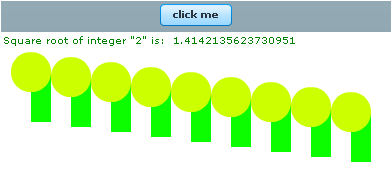Ads

Tutorials

# Action Script 'include' statement example

Example below shows two consequent action script files: GeometricalFigures.as and Sqrt.as, and the third example, i.e., Figure.mxml in our main mxml file inside which the working of 'include' statement has been demonstrated.

Example below shows two consequent action script files: GeometricalFigures.as and Sqrt.as, and the third example, i.e., Figure.mxml in our main mxml file inside which the working of 'include' statement has been demonstrated.

# Action Script 'include' statement example

Example below shows two consequent action script files: GeometricalFigures.as and Sqrt.as, and the third example, i.e. Figure.mxml is our main flex file inside which the working of  include statement has been demonstrated. Above mentioned action script files are in the includes folder that has been called by include statement in the main mxml file. The root directory of includes folder should match with the root directory and folders of your main mxml file and ant build file, here it is obvious that your build file and mxml file have same root directory. Now in the main mxml file, <mx:Script> tags  are made in which two include statements are used to call two action script files. Action script files posssess .as extension. For calling the files their relative paths are used.

Syntax for using include statement:-

include '  ';

Action script files can also be called through the source attribute property in <mx:Script> tag, but in that case only a single action script file will be called.
Noto : The source property and include statement cannot be used together in <mx:Script> tags.

Syntax for using 'source' attribute:-

<mx:Script source  =  '  '> ...... </mx:Script>

In Our first action script file, GeometricalFigures.as two packages flash.dispaly and mx.core has been imported for getting the instances of Sprite and UIComponent classes. Further in the code, two geometrical figures have been created and for creating these figures two Sprite type variables have been created.  Now graphics feature of Sprite class provides two static methods: .beginFill() and .drawCircle/Rect(), and among these methods the first one fills color in the figure and the second one creates the figure. Now our Sprite variables that we have created are called inside the UIComponent variable through .addChild function, now the UIComponent variable is called inside the flex Canvas container through the container's  id  property, which means figures will form inside the Canvas.

GeometricalFigures.as

 ``` import flash.display.Sprite;   import mx.core.UIComponent;   public function sqrt():void{   var num:Number = Number(Math.sqrt(2));   bn.text = 'Square root of integer "2" is: ' + num.toString();   }   public var x1:Number = 30;   public var y1:Number = 40;   public var z1:Number = 20;   public var f1:Number = 50;   public function draw():void{   var circle:Sprite = new Sprite();   circle.graphics.beginFill(0xCCFF00);   circle.graphics.drawCircle(x1,y1,z1);   var rectangle:Sprite = new Sprite();   rectangle.graphics.beginFill(0xCFC00);   rectangle.graphics.drawRect(x1, y1, z1, f1);   var rose:UIComponent = new UIComponent();   rose.addChild(rectangle);   rose.addChild(circle);   canvas.addChild(rose);   x1 = x1 + 40;   y1 = y1 + 5;   z1 = z1 + 0;   f1 = f1 + 0   }```

Sqrt.as

 ``` public function sqrt():void{   var num:Number = Number(Math.sqrt(2));   bn.text = 'Square root of integer "2" is: ' + num.toString(); }```

Figures.mxml

 ```      include 'includes/GeometricalFigures.as';   include 'includes/Sqrt.as';                ```

Figures.swfDownload the code

Ads

Ads